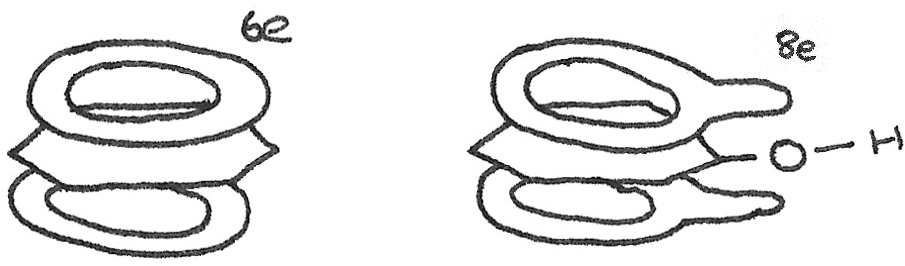# Benzene and Phenol

## Aromatic Hydrocarbons

An aromatic hydrocarbon, also known as an arene is a hydrocarbon containing at least one benzene ring. A benzene molecule has the molecular formula $C_{6}H_{6}$, or empirical formula $CH$, with the carbon atoms arranged in a cyclic ring. It can be drawn as a hexagon surrounding a circle.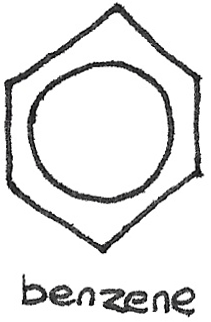When a hydrogen atom on the ring is substituted by another atom or group such as a halogen or an -ethyl group, a benzene derivative is formed.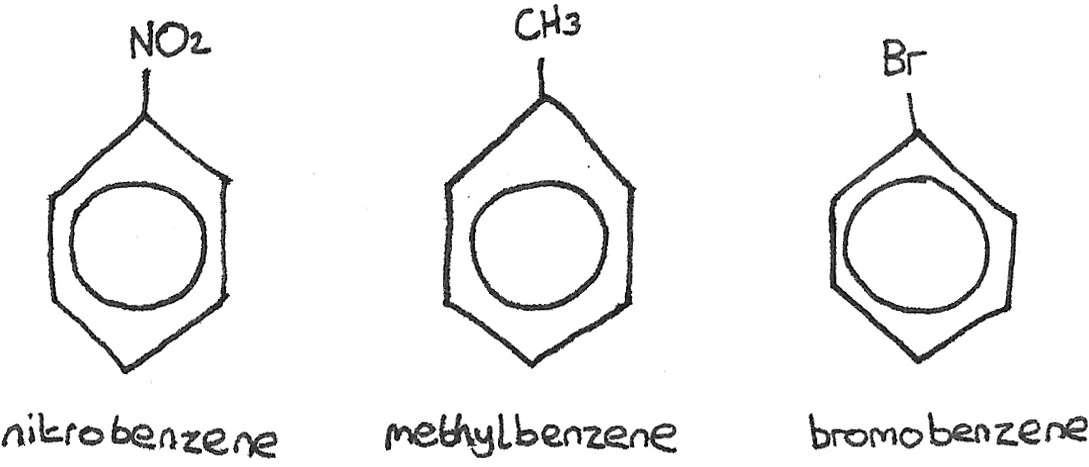If there are multiple functional groups, the carbon atoms in the benzene ring are numbered starting from the alkyl group, as is the case with 2-bromomethylbenzene.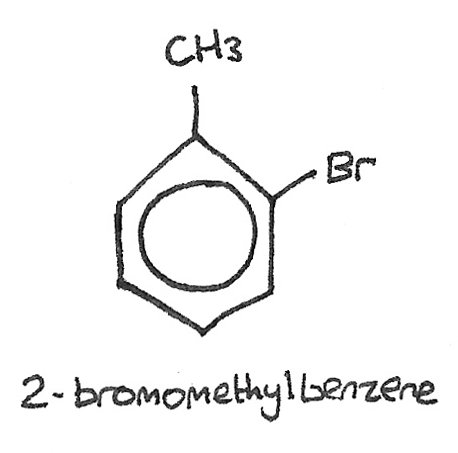### Synthesis

Benzene is often used in the synthesis of important aromatic compounds such as ethylbenzene, phenol and stryrene. Smaller quantities can be used in the distillation of alcohol and in explosives. Benzene is a colourless liquid with a sweet odour. It is also known to be a carcinogenic compound, as exposure can lead to an increase in the risk of cancer.

## Kekulé Structure

In the 1800s, the Kekulé structure was suggested for benzene with a six membered cyclic ring of carbon atoms, with alternate single and double bonds. The technical name of this structure is cyclohexa-1,3,5-triene.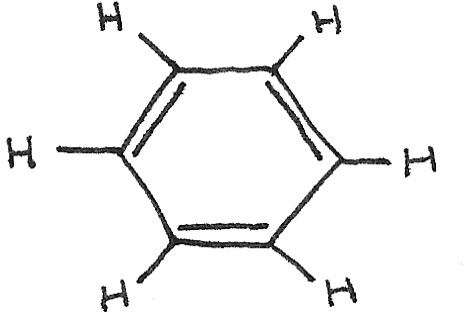This structure was found to be incorrect due to a number of unexpected physical and chemical properties.

### Low Reactivity

If $C=C$ double bonds were present then benzene would react in a similar way to alkenes, decolourising bromine water. However this does not happen as benzene doesn’t react with bromine. Benzene cannot react in any other electrophilic addition reactions.

### C-C Bond Lengths

The Kekulé structure contains three single $C-C$ bonds of length $0.153~nm$, and three double bonds of shorter length $0.134~nm$. This would result in an irregular shape. Observations with X-ray show that benzene actually has a regular hexagonal shape with six equal length ($0.139~nm$) carbon-carbon bonds.

### Hydrogenation of Benzene

For a single $C=C$ bond, the enthalpy change of hydrogenation ($\Delta_{\text{hydrogenation}}$) is $-120~kJmol^{-1}$. As the Kekulé structure has three $C=C$ bonds, it would be expected to have an $\Delta_{\text{hydrogenation}}$ of $-360~kJmol^{-1}$. Experimentally, it is shown to be much less at $-208~kJmol^{-1}$. This means that the real structure of benzene is $152~kJmol^{-1}$ more stable than the Kekulé structure. This energy is known as the delocalisation energy.

## Delocalised Electron Model

#### Delocalised Electrons

Electrons that are shared between more than one atom.

The failings in the Kekulé structure lead to the development of the delocalised model for benzene.

This structure has a hexagonal planar ring shape with six carbon atoms. Around each carbon atom, the bond angles are $120˚$, in a trigonal planar shape. Each carbon atom is joined to two other carbon atoms and one hydrogen atom through sigma ($\sigma$) bonds. A fourth electron in a $2p$ orbital, stays above and below the atom. Neighbouring $p$ orbital electrons overlap forming a system of $\pi$ bonds, with a delocalised electron ring structure.### Chemical Reactivity of Benzene

The increased stability of benzene makes addition reactions difficult. Some reactions that do not occur with benzene include:

• Decolorisation of bromine water.
• Reactions with strong acids ($HNO_{3}$).
• Reactions with halogens ($Cl_{2}$, $Br_{2}$).

This is because addition reactions would require the delocalised ring structure to be broken, reducing the stability of the molecule. Substitution reactions are therefore more favourable.

## Reactions of Benzene

The regions of high electron density above and below the plane of the carbon atoms attract electrophiles, causing electrophilic substitution. An electrophile is an electron pair acceptor attracted to an area of high electron density.

### Nitration by Electrophilic Substitution

In this reaction, a bonded hydrogen atom is replaced by a $-NO_{2}$ group. Benzene can be reacted with concentrated nitric acid ($HNO_{3}$), with concentrated sulphuric acid ($H_{2}SO_{4}$) acting as a catalyst at a temperature of 50°.

$$C_{6}H_{6} + HNO_{3} \rightarrow C_{6}H_{5}NO_{2} + H_{2}O$$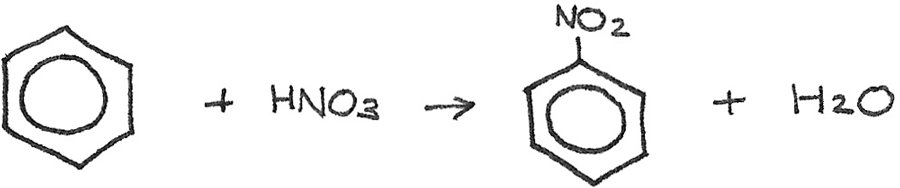The sulphuric acid is used to generate an electrophile, which is the nitronium ion ($NO_2^{+}$) from the nitric acid. The reaction is:

$$H_{2}SO_{4} + HNO_{3} \rightarrow HSO_{4}^{-} + NO_{2}^{+} + H_{2}O$$

The mechanism for the nitration of benzene is: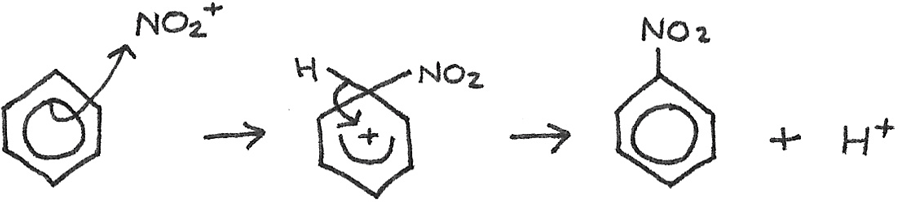The curly arrows are used to indicate the movement of an electron pair in a reaction mechanism. The $H^{+}$ ion then reacts with the $HSO_{4}^{-}$ to reform the $H_{2}SO_{4}$.

### Halogenation

Benzene will only react with halogens in the presence of a halogen carrier, such as $FeBr_{3}$ or $AlBr_{3}$ for bromine. The halogen carrier acts as a catalyst. The reaction is as follows: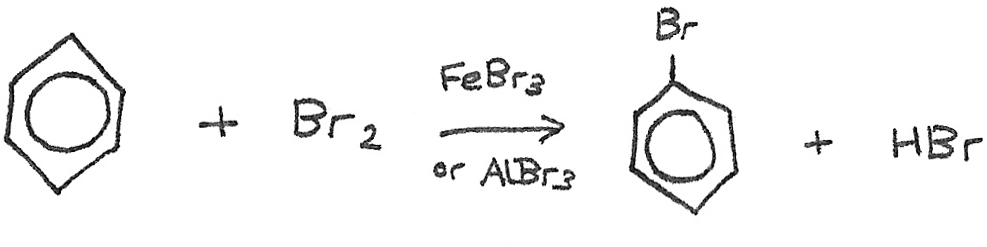The $Br^{+}$ ion is formed as below:
$$Br_{2} + FeBr_{3} \rightarrow Br^{+} + FeBr_{4}^{-}$$

The electrophile now reacts with the benzene in the following mechanism: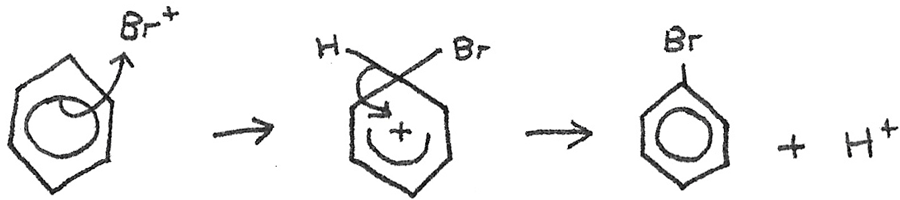The $H^{+}$ now reacts with the $FeBr_{4}^{-}$ to reform $HBr$ and $FeBr_{3}$.

$$H^{+} + FeBr_{4}^{-} \rightarrow FeBr_{3} + HBr$$

### Reactivity of Cyclohexene and Benzene

Cyclohexene reacts like a typical alkene, with the bromine atoms added across the double bond in an electrophilic addition reaction.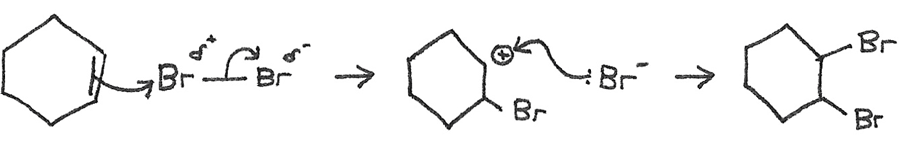However when bromine is added to benzene, the bromine remains orange and there is no reaction. This is because:

• Benzene has delocalised electrons spread over 6 carbon atoms, whereas alkenes have localised electrons above and below the 2 carbon atoms in the double bond.
• Benzene has a lower $\pi$ electron density resulting in an insufficient electron density to polarise the $Br_{2}$ molecule. This results in the requirement of a halogen carrier.

## Phenol

The phenols are a group of organic compounds in which a hydroxyl group ($-OH$) is attached directly to a benzene ring. Phenol is the simplest of the phenols, with one of the attached hydrogen atoms on a benzene ring replaced with a $-OH$ group. It is a slightly soluble grey solid, with the solubility due to the polar $-OH$ group, able to form hydrogen bonds with the water molecules.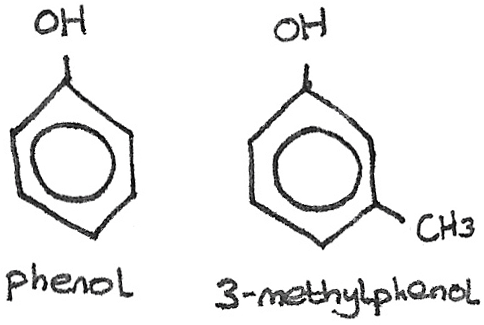In everyday use, phenol can be used in detergents, antiseptics and aspirin.

## Reactions of Phenol

#### Reaction with Sodium Hydroxide

When phenol is dissolved in water, it forms a weak acidic solution by losing the $H^{+}$ ion from the $-OH$ group.

$$C_{6}H_{5}OH + \text{aq} \leftrightharpoons C_{6}H_{5}O^{-} + H^{+}$$

Phenol can be neutralised by aqueous sodium hydroxide to form the salt sodium phenoxide, $C_{6}H_{5}O^{-}Na^{+}$ and water:

$$C_{6}H_{5}OH + NaOH \rightarrow C_{6}H_{5}O^{-}Na^{+} + H_{2}O$$

#### Reaction with Sodium

When a reactive metal such as sodium is added to phenol, the metal fizzes producing hydrogen gas. The salt $C_{6}H_{5}O^{-}Na^{+}$ is produced.

$$2C_{6}H_{5}OH + 2Na \rightarrow 2C_{6}H_{5}O^{-}Na^{+} + H_{2}$$

#### Reaction with Bromine

Unlike benzene, phenol is able to undergo electrophilic substitution with halogens at room temperature, without the need for a halogen carrier. When bromine is added to aqueous phenol, a white precipitate of 2,4,6-tribromophenol is formed.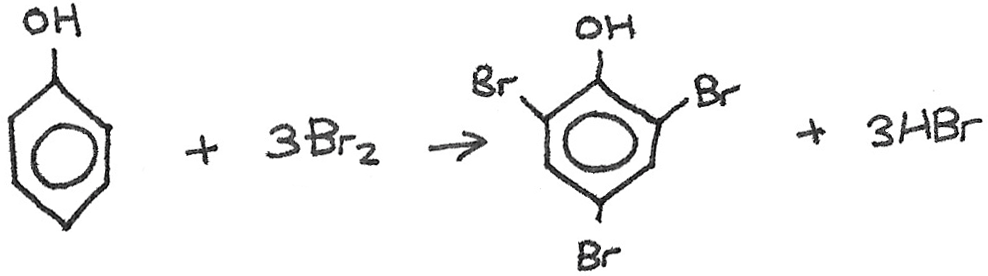The increased reactivity compared to benzene is due to the lone pair of p-orbital electrons on the oxygen atom in the phenol group. The electron pair is delocalised into the ring structure, activating the ring. This increases the electron density of the molecule, which induces dipoles in the bromine molecule. Electrophilic substitution can then take place.